AP Physics C: Mechanics : Using Torque Equations

Example Questions

2 Next →

Example Question #11 : Circular And Rotational Motion

An art sculpture comprised of a long hollow painted cylinder is designed to pivot about an axis located along its long edge. The cylinder has mass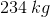, radius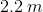, and length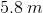.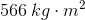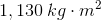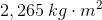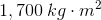Explanation:

The moment of inertia for a hollow cylinder about its center is given by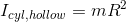The parallel axis is applied here because the pivot axis is located on the outside edge of the cylinder, a distancefrom the center of mass: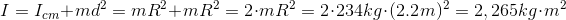Incidentally, for this problem, the length does not contribute to the moment of inertia.

Example Question #21 : Circular And Rotational Motion

A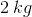meter stick is nailed to a table at one end and is free to rotate in a horizontal plane parallel to the top of the table. A force of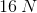is applied perpendicularly to its length at a distance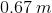from the nailed end.

Calculate the resulting angular acceleration experienced by the meter stick.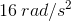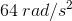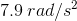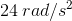Explanation:

For a net torque applied to an object free to rotate,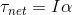The net torque for this problem is supplied by theforce applied at a distancefrom the pivot. The torque of a force is calculated by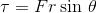The moment of inertia for a long uniform thin rod about its end is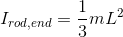Combining above,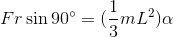Solving for the angular acceleration,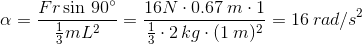Example Question #22 : Circular And Rotational Motion

Ameter stick is secured at one end so that it is free to rotate in a vertical circle. It is held perfectly horizontally, and released.

Calculate the instantaneous angular acceleration the moment it is released.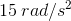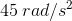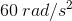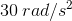Explanation:

For a net torque applied to an object free to rotate,The net torque for this problem is supplied by theforce applied at a distancefrom the pivot. The torque of a force is calculated byThe moment of inertia for a long uniform thin rod about its end isCombining above,where the force causing the torque is the meter stick's weight, which is applied at the center of mass of the meter stick - a distance of half the length away from its end.

Solving for the angular acceleration,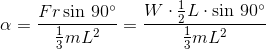Solving numerically,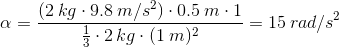Example Question #23 : Circular And Rotational Motion

A 0.18 m long wrench is used to turn the nut on the end of a bolt. A force of 85 N is applied downward to the end of the wrench, as shown in the figure. The angle between the force and the handle of the wrench is 65 degrees.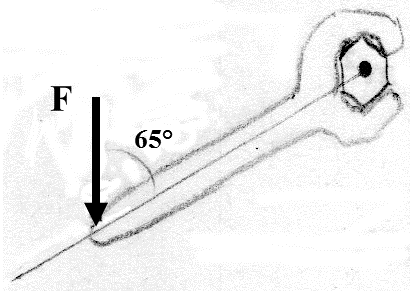What is the magnitude and direction of the torque (around the center of the bolt) due to this force?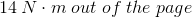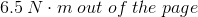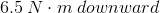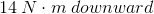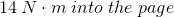Explanation:

To calculate the magnitude of the torque,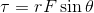where the radiusis the distance between the center of rotation and the location of the forceand the angle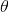is between the radiusand the force.

The magnitude is thus,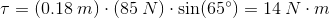The direction of torque is perpendicular to the plane of the radiusand the force, and is given by Right Hand Rule by crossing the radius vector into the force vector. For this situation, the radius vector is left and downward and the force is downward, resulting in the direction of the torque out of the page.

2 Next →